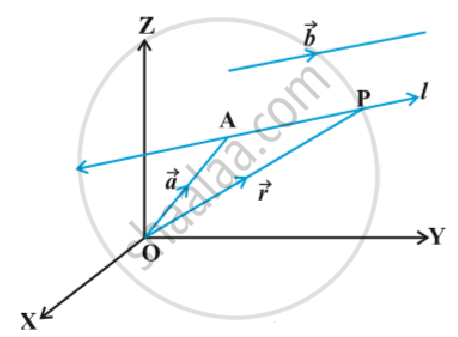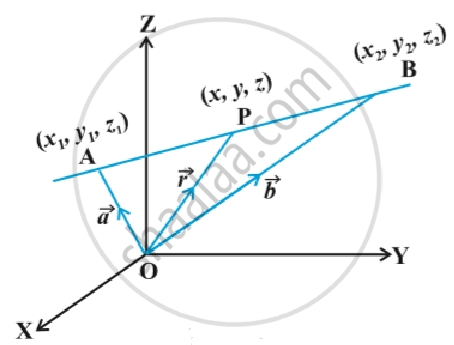Equation of a Line in Space

notes

Equation of a line through a given point and parallel to a given vector vec b:
Let vec a be the position vector of the given point A with respect to the origin O of the rectangular coordinate system. Let l be the line which passes through the point A and is parallel to a given vector vec b .
Let  vec r be the position vector of an arbitrary point P on the line in following fig.Then vec (AP)  is parallel to the vector vec b , i.e., vec (AP) = λ vec b , where λ is some real number.
But  vec (AP) = vec (OP) - vec (OA)
i.e. lambda vec b =  vec r - vec a
Conversely, for each value of the parameter λ, this equation gives the position vector of a point P on the line.  Hence, the vector equation of the line is given by
vec r = vec a + lambda vec b    ...(1)

Remark:  If vec b = a hat i + b hat j + c hat k  , then a,b ,c are direction ratios of the line and conversely , if a, b , c are direction ratios of a line , then vec b = a hat i +b hat j +c hat k  will be the parallel to the line. Here, b should not be confused with | vec b|.

Derivation of cartesian form from vector form
Let the coordinates of the given point A be (x_1, y_1, z_1) and the direction  ratios of the line be a, b, c. Consider the coordinates of any point P be (x, y, z). Then
vec r = x hat i + y hat j + z hat k ;
vec a = x_1 hat i + y_1 hat j + z_1 hat k
and vec b = a hat i + b hat j + c hat k
Substituting these values in (1) and equating the coefficients of hat i , hat j " and " hat k , we get
x = x_1 + lambda a ; y = y_1 +lambda  b ; z = z_1 + lambda c  ...(2)
These are parametric equations of the line. Eliminating the parameter λ from (2), we get

(x - x_1)/a = (y - y_1)/a = (z - z_1)/c      ...(3)
This is the Cartesian equation of the line.

Equation of a line passing through two given points:
Let vec a and vec b be the position vectors of two points A(x_1 , y_1 , z_1) and B (x_2 , y_2 , z_2) respectively that are lying on a line in following fig.Let vec rbe the position vectors of an arbitrary point P(x , y , z), then P is a point on the  line if and only if vec (AP) = vec r - vec a and   vec (AB) = vec b - vec a are collinear vectors. Therefore, P is on the line if and only if vec r = vec a = lambda (vec b - vec a)
or vec r = vec a + lambda (vec b - vec a) , lambda ∈ R   ...(1)
This is the vector equation of the line.

Derivation of cartesian form from vector form
We have
vec r = x hat i + y hat j + z hat k , vec a = x_1 hat i +y_1 hat j + z_1 hat k and vec b = x_2 hat i + y_2 hat j + z_2 hat k,
Substituting these values in (1), we get
x hat i + y hat j+ z hat k = x_1hat i +y_1 hat j + z_1 hat k , + lambda [(x_2 - x_1) hat i +(y_2 - y_1) hat j + (z_2 -z_1 hat k)]
Equating the like coefficients of hat i , hat j , hat k ,we have
x = x_1 + lambda (x_2 - x_1); y = y_1 + lambda (y_2-y_1) ; z = z_1 +lambda (z_2 - z_1)
On eliminating  λ, we obtain

(x - x_1)/(x_2 - x_1) = (y - y_1)/ (y_2 - y_1) = (z - z_1) / (z_2 - z_1)

which is the equation of the line in Cartesian form.

If you would like to contribute notes or other learning material, please submit them using the button below.

Shaalaa.com

Three Dimensional Geometry Part 2 -Straight Line [00:21:23]
S
0%